# Texas Go Math Grade 4 Lesson 14.3 Answer Key Measure and Draw Angles

Refer to our Texas Go Math Grade 4 Answer Key Pdf to score good marks in the exams. Test yourself by practicing the problems from Texas Go Math Grade 4 Lesson 14.3 Answer Key Measure and Draw Angles.

## Texas Go Math Grade 4 Lesson 14.3 Answer Key Measure and Draw Angles

Essential Question

How can you use a protractor to measure and draw angles?
We will use a protractor by placing the midpoint of the protractor on the vertex of the angle and then we will mark the angle which we needed.

Unlock the Problem

Emma wants to make a clay sculpture of her daughter as she appears in the photo from her dance recital. How can she measure ∠DOE, or the angle formed by her daughter’s arms?

A protractor is a tool for measuring the size of an angle.Activity Measure ∠DCE using a protractor.

Materials

• protractor

STEP I Place the center point of the protractor on vertex C of the angle.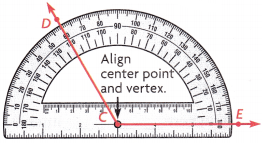STEP 2 Align the 0° mark on the scale of the protractor with ray CE.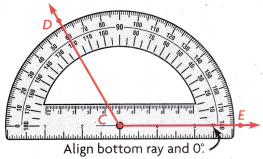STEP 3 Find where ray CD intersects the same scale. Read the angle measure on that scale. Extend the ray if you need to.
The m∠DCE = _________ . Read the m∠DCE as the “measure of angle DCE”.
So, the angle formed by Emma’s daughters arms is ____________ .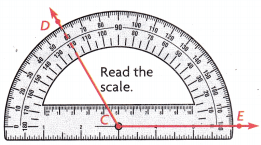The angle formed by Emma’s daughter’s arms is 120 degrees.

Explanation:
Here, the m∠DCE is 120 degrees. So the angle formed by Emma’s daughter’s arms is 120 degrees.

Activity Draw ∠KLM with a measure of 82°

Materials

• protractor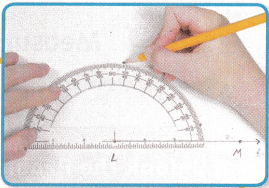STEP 1 Use the straight edge of the protractor to draw and label ray LM.

STEP 2 Place the center point of the protractor on point L.
Align ray LM with the 00 mark on the protractor.

STEP 3 Using the same scale, mark a point at 82°. Label the point K.

STEP 4 Use the straight edge of the protractor to draw ray LK.

Share and Show

Question 1.
Measure ∠ABC.
Place the center of the protractor on point ___________ .
Align ray BC with ___________.
Read where ___________ intersects the same scale.
So, the m∠ABC is ___________.The m∠ABC is 115 degrees.

Explanation:
Here, we will place the center of the protractor on point B and align ray BC with AB then we will read where m∠ABC intersects the same scale. So, the m∠ABC is 115 degrees.

ERROR Alert: Be sure to use the correct scale on the protractor. Ask yourself: Is the measure reasonable?

Lesson 14.3 Answer Key Measure and Draw Angles Question 2.m∠ONM = ____________
m∠ONM = 55°.

Explanation:
The angle of m∠ONM  is 55°.

Question 3.m∠TSR = ____________
m∠TSR = 105°.

Explanation:
The angle of m∠TSR is 105°.

Use a protractor to draw the angle.

Question 4.
170°
The angle of 170° is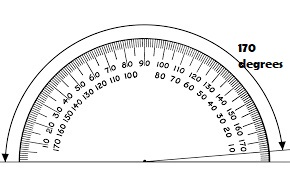Question 5.
78°
The angle of 78° is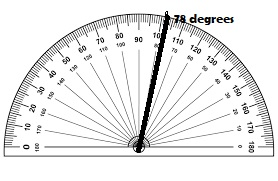Math Talk

Mathematical Processes
Describe how drawing and measuring angles are similar.

Problem Solving

Question 6.
Representations Mrs. Murphy is building a wheelchair ramp outside her business. The angle of the ramp should be 5°. Draw a picture in the space to the right to show a model of the ramp.
Given that Mrs. Murphy is building a wheelchair ramp outside her business and the angle of the ramp should be 5°. So the picture is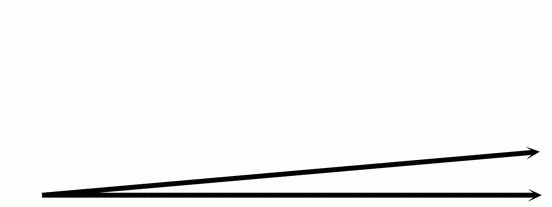Question 7.H.O.T. Write Math Draw an angle with a measure of 0°. Describe your drawing.

Grade 4 Measure and Draw Angles Lesson 11.3 Question 8.
H.O.T. What’s the Error? Tracy measured an angle as 50° that was actually 130°. Explain her error.

Use the diagrams and a protractor for 9-11.

Question 9.
Use Tools In the Northern Hemisphere, Earth’s axis is tilted away from the sun on the first day of winter, which is often on December 21. What is the measure of the marked angle on the first day of winter, the shortest day of the year?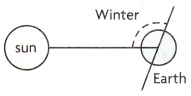Question 10.
Use Diagrams Earth’s axis is not tilted away from or toward the sun on the first days of spring and fall, which are often on March 20 and September 22. What is the measure of the marked angle on the first day of spring or fall?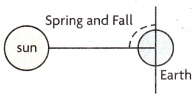Question 11.
In the Northern Hemisphere, Earth’s axis is tilted toward the sun on the first day of summer, which is often on June 21. What is the measure of the marked angle on the first day of summer, the longest day of the year?Question 12.
Kate tosses a stone in the water at a stone-skipping tournament. It forms a 200 angle with the water and skips many times. Which angle measures 20°?
(A)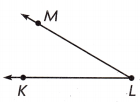(B)(C)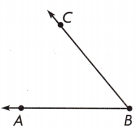(D)B.

Explanation:
The angle that measures 20° is option B.

Question 13.
Kyle moves furniture up a ramp to get it on a truck. What is the measure of the angle formed by the ramp?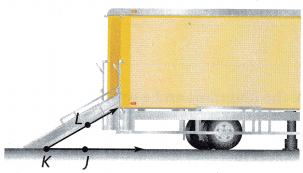(A) 150°
(B) 80°
(C) 300
(D) 60°
D.

Explanation:
The measure of the angle formed by the ramp is 60°.

Multi-Step Maria builds two sets of stairs in her doll house. Measure the angles in the picture at the right. What is the difference between the measures of the angles formed by the stairs?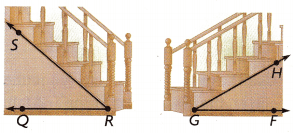(A) 1°
(B) 30°
(C) 40°
(D) 10°
B.

Explanation:
Here, the angle <QRS is 60°, and the <HGF is 30°. So the difference between them is 60°-30° which is 30°.

TEXAS Test Prep

Question 15.
What is the measure of LQRS?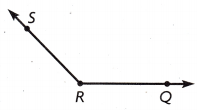(A) 35°
(B) 45°
(C) 135°
(D) 155°

C.

Explanation:
The measure of <QRS is 135°.

### Texas Go Math Grade 4 Lesson 14.3 Homework and Practice Answer Key

Use a protractor to find the angle measure.

Question 1.m∠ABC = ___________
m∠ABC =110°.

Explanation:
By measuring the angle with a protractor we will get the angle m∠ABC as 110°.

Question 2.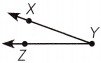m∠XYZ = __________
m∠XYZ + 20°.

Explanation:
By measuring the angle with a protractor we will get the angle m∠XYZ as 20°.

Use a protractor to find the angle measure.

Question 3.
125°
The angle of 125° is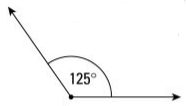Question 4.
86°
The angle of 86° is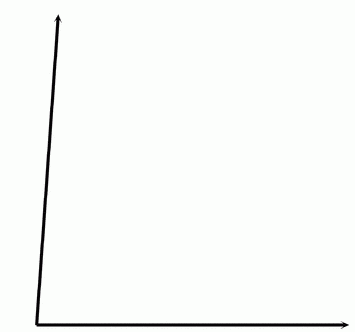Question 5.
35°
The angle of 35° is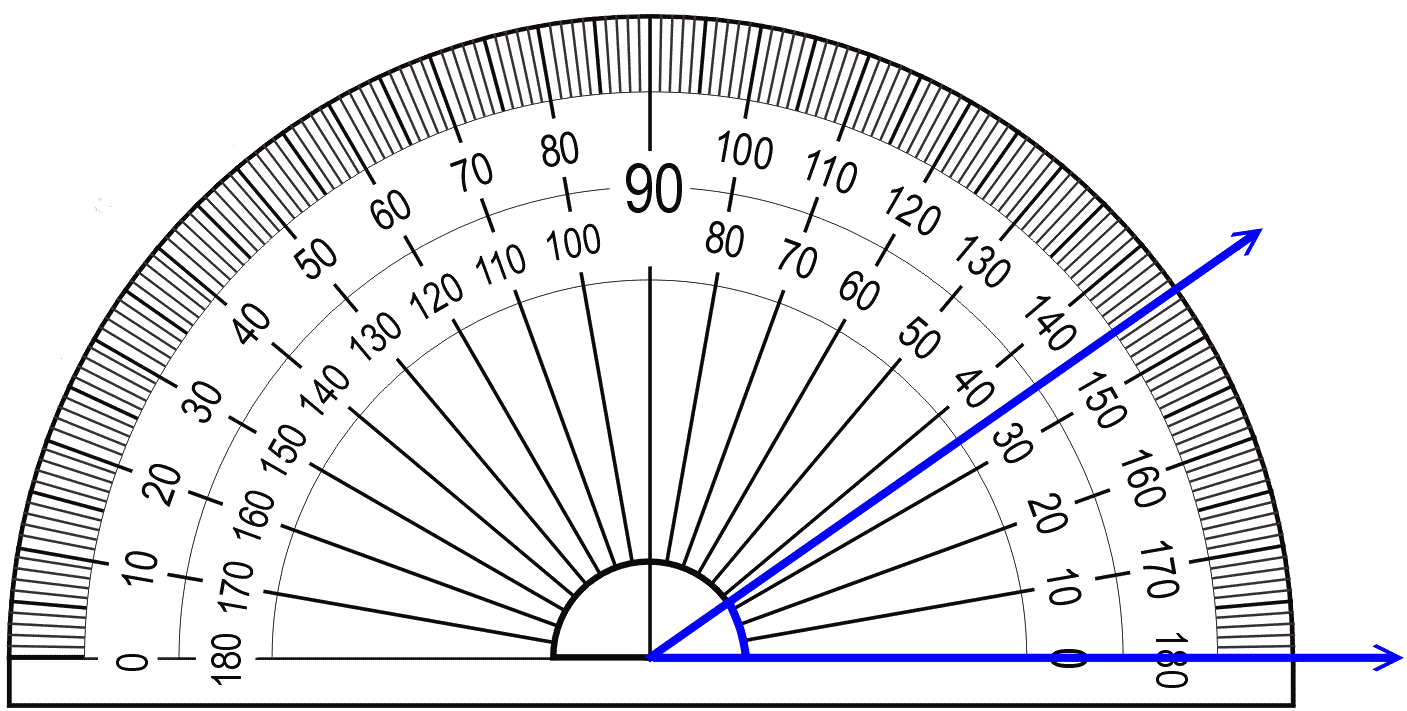Question 6.
180°
The angle of 180° is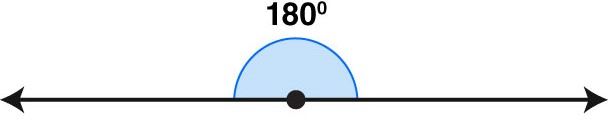Problem Solving

Question 7.
George leaned a ladder against his house. The angle of the ladder to the ground measured 65°. Draw a picture to show a model of the angle.
Given that George leaned a ladder against his house and the angle of the ladder to the ground measured 65°, so the picture to show a model of the angle is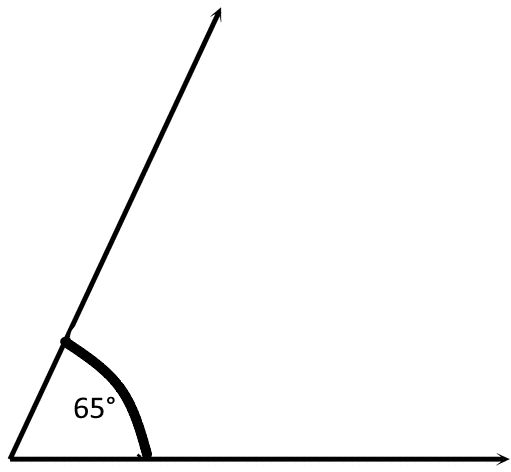Grayson built a skateboard ramp. The angle of the ramp is 14°. Draw a picture to show a model of the ramp.
Given that Grayson built a skateboard ramp and the angle of the ramp is 14°, the model of the ramp is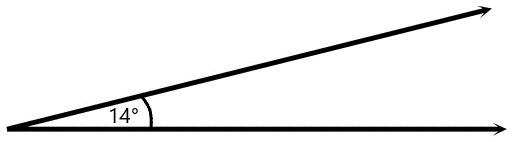Lesson Check

Question 9.
What is the measure of ∠LMN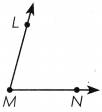(A) 110°
(B) 70°
(C) 105°
(D) 75°
D.

Explanation:
The measure of ∠LMN is 75°.

Question 10.
Whitney drew these angles. Which of the angles has a measure of 130°?
(A)(B)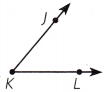(C)(D)B.

Explanation:
The angle that measures 130° is B.

Question 11.
An artist is cutting glass triangles to make a star-shaped stained glass window. What is the measure of each of the angles formed at the tips of the star?(A) 140°
(B) 30°
(C) 40°
(D) 110°
B.

Explanation:
The measure of each of the angles formed at the tips of the star is 30°.

Question 12.
Multi-Step Elizabeth is making a quilt with scraps of fabric. Measure the angles in the picture. What is the difference between the measures of the angles of each scrap of fabric?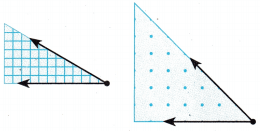(A) 25°
(B) 15°
(C) 165°
(D) 75°
A.

Explanation:
The measure of the angles of each scrap of fabric is 25°.

Question 13.
Hector drew a map of his Street corner. Use a protractor to find x, the measure of an angle formed where Jefferson Street intersects Washington Street.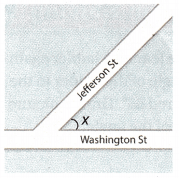(A) 130°
(B) 135°
(C) 45°
(D) 62°
C.

Explanation:
The measure of an angle formed where Jefferson Street intersects Washington Street is 45°.

Question 14.
Multi-Step After eating at a restaurant, Faith had one quarter of her pizza left to take home. She took the pizza home and cut it into three equal slices. What is the angle measure of each of the slices of pizza?
(A) 60°
(B) 40°
(C) 90°
(D) 30°